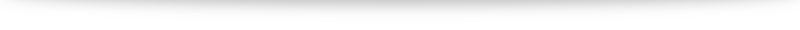# Is $\sqrt{x^2} = x$? The many different modulus functions.

What is $\sqrt{x^2}$? Most of us will intuitive say "$x$": after all, $\sqrt{9} = \sqrt{3^2} = \sqrt{3}$, for example. However, what is $\sqrt{(-3)^2}$?

It is not $-3$ and is in fact $\sqrt{(-3)^2} = \sqrt{9} = 3$. Hence $\sqrt{x^2} =x$ is only valid if $x$ is non-negative. If $x$ is negative, it turns out that $\sqrt{x^2} = -x$.

The reason for this stems from definition: the symbol $\sqrt{ \cdot}$ is defined to be the "positive square root" when there are actually two possible square roots to every positive real number (this is the reason the equation $x^2 = k$ has two solutions, $\pm \sqrt{k}$, for positive $k$).

A compact way to summarize:

This brings us to the modulus function. For real numbers $x$, we can think of $|x|$ as "making $x$ positive/non-negative". This can be defined using a piecewise function: $$|x| = \begin{cases} x \quad &\textrm{if } x \geq 0 \\ -x \quad &\textrm{if } x < 0 \end{cases}$$

We see the modulus in two other topics in our syllabus: complex numbers and vectors.
For a complex number $z = x+yi$, the modulus $|x+yi| = \sqrt{x^2+y^2}$ measures the distance between the point representing $z$ and the origin on an Argand diagram. For a 3-dimensional vector, we define the magnitude of a vector to be $\left |\begin{pmatrix} x \\ y \\ z \end{pmatrix} \right | = \sqrt{x^2+y^2+z^2}$.

To summarize:

This justifies the use of the same symbol. In the context of each topic, the use of the modulus is used to convey slightly different ideas. From an abstract point of view, however, the different modulus we have encountered aren't that different. This idea generalizes to $n$-dimensions and is called the Euclidean norm (Euclidean after the Greek mathematician Euclid, since the formula is an extension of Pythagoras' Theorem).